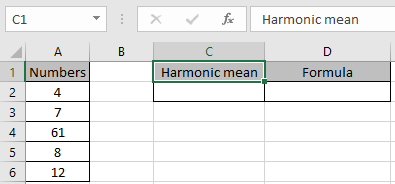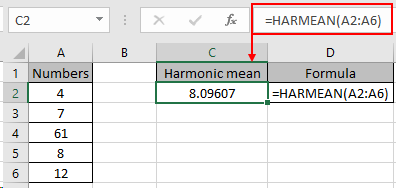# How to use the HARMEAN Function in Excel

Harmonic mean of n numbers (a ,b ,c ,d , . . .) in mathematics is calculated using the below formula.

Harmonic mean = n/(1/a + 1/b + 1/c + 1/d + . . .)

In excel we have HARMEAN function to calculate the Harmonic mean of the values.

The HARMEAN function is a mathematical function used to calculate the Harmonic mean of the numbers.

Syntax:

=HARMEAN (number1, [number2], ...)

Note: This Excel harmonic mean formula ignores text, empty cells and logical_values.

Let’s understand this function using it as an example.Use the formula to get Harmonic mean of the values.

=HARMEAN(A2:A6)

A2 : A6 :- values to the function are provided using the cell reference.As you can see, the function returns the Harmonic mean of the values which comes out to be 8.09607 .
Notes:

1. The function return #NUM! Error if any negative number is supplied.
2. All input values except numbers are ignored by the function.

Hope you understood how to use Harmonic mean function and referring arrays in Excel. Explore more articles on Excel function here. Please feel free to state your query or feedback for the above article.

Related Articles
How to use the DCOUNT function in Excel

How to use the GEOMEAN function in Excel

How to use Wildcards in Excel

Popular Articles

Edit a dropdown list

If with conditional formatting

Vlookup by date

Terms and Conditions of use

The applications/code on this site are distributed as is and without warranties or liability. In no event shall the owner of the copyrights, or the authors of the applications/code be liable for any loss of profit, any problems or any damage resulting from the use or evaluation of the applications/code.